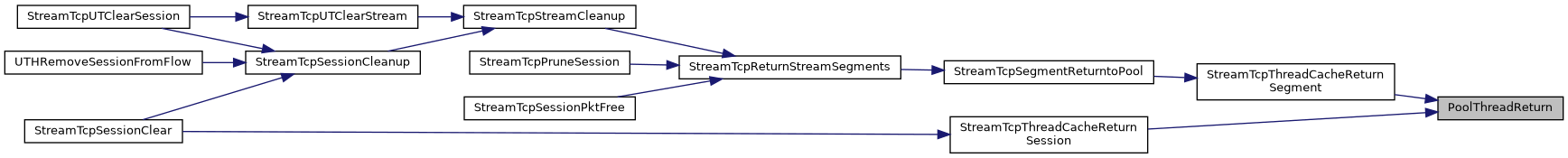suricata
Pool

Pool are an effective way to maintain a set of ready to use structures. More...

## Files

file  util-pool.c

file  util-pool.h

## Functions

PoolThreadPoolThreadInit (int threads, uint32_t size, uint32_t prealloc_size, uint32_t elt_size, void *(*Alloc)(void), int(*Init)(void *, void *), void *InitData, void(*Cleanup)(void *), void(*Free)(void *))
per thread Pool, initialization function More...

expand pool by one for a new thread More...

return data to thread pool More...

PoolPoolInit (uint32_t size, uint32_t prealloc_size, uint32_t elt_size, void *(*Alloc)(void), int(*Init)(void *, void *), void *InitData, void(*Cleanup)(void *), void(*Free)(void *))
Init a Pool. More...

void PoolFree (Pool *p)

void PoolPrint (Pool *p)

void * PoolGet (Pool *p)

void PoolReturn (Pool *p, void *data)

void PoolPrintSaturation (Pool *p)

void PoolRegisterTests (void)

## Detailed Description

Pool are an effective way to maintain a set of ready to use structures.

To create a Pool, you need to use PoolInit(). You can get an item from the Pool by using PoolGet(). When you're done with it call PoolReturn(). To destroy the Pool, call PoolFree(), it will free all used memory.

## ◆ PoolFree()

 void PoolFree ( Pool * p )

Definition at line 220 of file util-pool.c.

References Pool_::alloc_stack, Pool_::Cleanup, PoolBucket_::data, and PoolBucket_::next.

Here is the caller graph for this function: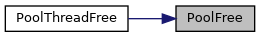## ◆ PoolGet()

 void* PoolGet ( Pool * p )

Definition at line 275 of file util-pool.c.

Here is the caller graph for this function: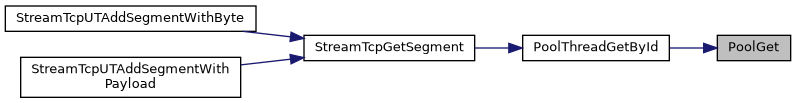## ◆ PoolInit()

 Pool* PoolInit ( uint32_t size, uint32_t prealloc_size, uint32_t elt_size, void *(*)(void) Alloc, int(*)(void *, void *) Init, void * InitData, void(*)(void *) Cleanup, void(*)(void *) Free )

Init a Pool.

PoolInit() creates a Pool. The Alloc function must only do allocation stuff. The Cleanup function must not try to free the PoolBucket::data. This is done by the Pool management system.

Parameters
 size prealloc_size elt_size Memory size of an element Alloc An allocation function or NULL to use a standard SCMalloc Init An init function or NULL to use a standard memset to 0 InitData Init data Cleanup a free function or NULL if no special treatment is needed Free free func
Return values
 the allocated Pool

Definition at line 84 of file util-pool.c.

Here is the caller graph for this function:## ◆ PoolPrint()

 void PoolPrint ( Pool * p )

Definition at line 268 of file util-pool.c.

References Pool_::alloc_stack_size, and Pool_::empty_stack_size.

## ◆ PoolPrintSaturation()

 void PoolPrintSaturation ( Pool * p )

Definition at line 373 of file util-pool.c.

References Pool_::max_buckets, Pool_::outstanding, and SCLogDebug.

## ◆ PoolRegisterTests()

 void PoolRegisterTests ( void )

Definition at line 715 of file util-pool.c.

References UtRegisterTest().

Here is the call graph for this function: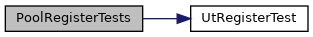## ◆ PoolReturn()

 void PoolReturn ( Pool * p, void * data )

Definition at line 333 of file util-pool.c.

Here is the caller graph for this function: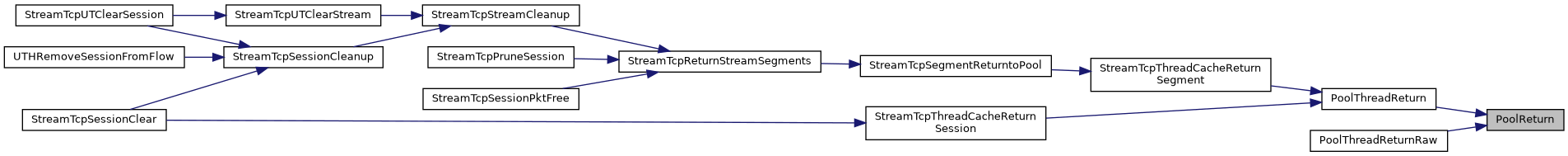expand pool by one for a new thread

grow a thread pool by one

Return values

Definition at line 92 of file util-pool-thread.c.

Here is the call graph for this function: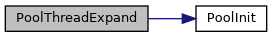Note
wrapper around PoolFree()
Parameters

Definition at line 150 of file util-pool-thread.c.

Here is the call graph for this function: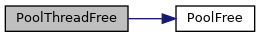Here is the caller graph for this function:Note
wrapper around PoolGet()
Parameters
Return values
 ptr data or NULL

Definition at line 168 of file util-pool-thread.c.

Referenced by StreamTcpGetSegment().

Here is the call graph for this function: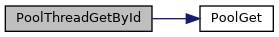Here is the caller graph for this function: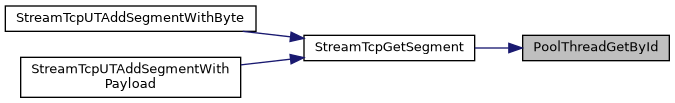PoolThread* PoolThreadInit ( int threads, uint32_t size, uint32_t prealloc_size, uint32_t elt_size, void *(*)(void) Alloc, int(*)(void *, void *) Init, void * InitData, void(*)(void *) Cleanup, void(*)(void *) Free )

Parameters

Definition at line 43 of file util-pool-thread.c.

Here is the call graph for this function:Definition at line 375 of file util-pool-thread.c.

References UtRegisterTest().

Here is the call graph for this function: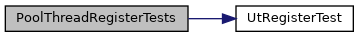Note
wrapper around PoolReturn()
Parameters
 pt thread pool data memory block to return, with PoolThreadReserved as it's first member

Definition at line 187 of file util-pool-thread.c.

Referenced by StreamTcpSegmentReturntoPool(), and StreamTcpSessionClear().

Here is the call graph for this function: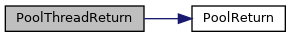Here is the caller graph for this function: